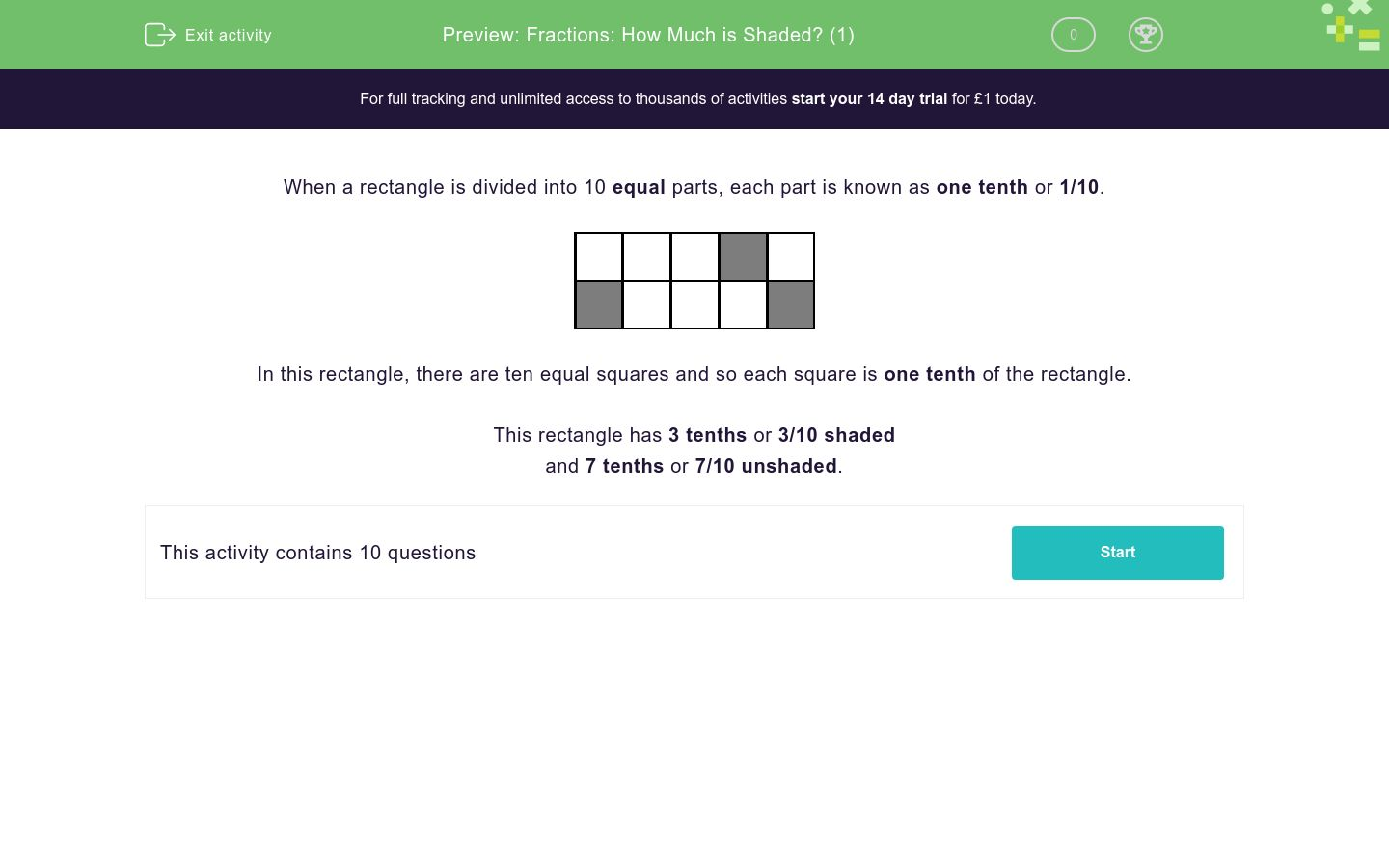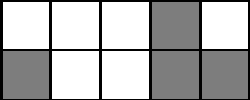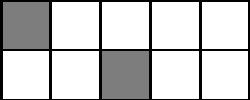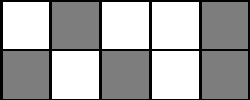# Fractions: How Much is Shaded? (1)

In this worksheet, students must count the number of tenths that have been shaded in a rectangle.Key stage:  KS 2

Curriculum topic:   Number: Fractions

Curriculum subtopic:   Count in Tenths

Difficulty level:### QUESTION 1 of 10

When a rectangle is divided into 10 equal parts, each part is known as one tenth or 1/10.In this rectangle, there are ten equal squares and so each square is one tenth of the rectangle.

This rectangle has 3 tenths or 3/10 shaded

and 7 tenths or 7/10 unshaded.

What fraction of the rectangle has been shaded?

Select how many tenths.6/10

4/10

2/10

What fraction of the rectangle has been shaded?

Select how many tenths.4/10

10/5

5/10

What fraction of the rectangle has been shaded?

Select how many tenths.8/10

2/10

4/10

What fraction of the rectangle has been shaded?

Select how many tenths.6/10

4/10

10/6

What fraction of the rectangle has been shaded?

Select how many tenths.8/10

2/10

4/10

What fraction of the rectangle has been shaded?

Select how many tenths.8/10

2/10

4/10

What fraction of the rectangle has been shaded?

Select how many tenths.3/10

7/10

10/7

What fraction of the rectangle has been shaded?

Select how many tenths.9/10

1/10

4/10

What fraction of the rectangle has been shaded?

Select how many tenths.4/10

3/10

6/10

What fraction of the rectangle has been shaded?

Select how many tenths.5/10

1/10

9/10

• Question 1

What fraction of the rectangle has been shaded?

Select how many tenths.6/10
EDDIE SAYS
6 of the 10 equal squares are shaded.
• Question 2

What fraction of the rectangle has been shaded?

Select how many tenths.5/10
EDDIE SAYS
5 of the 10 equal squares are shaded.
• Question 3

What fraction of the rectangle has been shaded?

Select how many tenths.8/10
EDDIE SAYS
8 of the 10 equal squares are shaded.
• Question 4

What fraction of the rectangle has been shaded?

Select how many tenths.4/10
EDDIE SAYS
4 of the 10 equal squares are shaded.
• Question 5

What fraction of the rectangle has been shaded?

Select how many tenths.2/10
EDDIE SAYS
2 of the 10 equal squares are shaded.
• Question 6

What fraction of the rectangle has been shaded?

Select how many tenths.2/10
EDDIE SAYS
2 of the 10 equal squares are shaded.
It doesn't matter which 2 squares are shaded.
• Question 7

What fraction of the rectangle has been shaded?

Select how many tenths.7/10
EDDIE SAYS
7 of the 10 equal squares are shaded.
• Question 8

What fraction of the rectangle has been shaded?

Select how many tenths.9/10
EDDIE SAYS
9 of the 10 equal squares are shaded.
• Question 9

What fraction of the rectangle has been shaded?

Select how many tenths.4/10
EDDIE SAYS
4 of the 10 equal squares are shaded.
• Question 10

What fraction of the rectangle has been shaded?

Select how many tenths.5/10
EDDIE SAYS
5 of the 10 equal squares are shaded.
This is the same as a half.
---- OR ----

Sign up for a £1 trial so you can track and measure your child's progress on this activity.

### What is EdPlace?

We're your National Curriculum aligned online education content provider helping each child succeed in English, maths and science from year 1 to GCSE. With an EdPlace account you’ll be able to track and measure progress, helping each child achieve their best. We build confidence and attainment by personalising each child’s learning at a level that suits them.

Get started